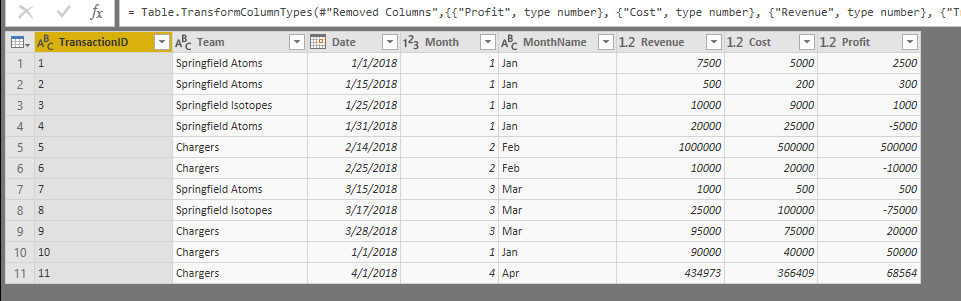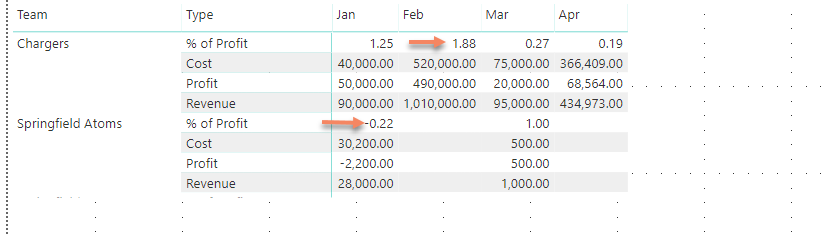cancel
Showing results for
Did you mean:Frequent Visitor

## How to calculate percentage

Hello,

I am attempting to recreate a Tableau dashboard and the data that was provided to me is in a pivot format. I've managed to unpivot the columns Revenue, Cost, Profit and % of Profit for the matrix table. The issue I am facing is that the column, % of profit is summing up rather than returning the single value for a given team and month.  Is there a way to prevent the % of profit from summing? Any advice / tips would be greatly appreciated.Original datanew measures for % of profitnew table to unpivot data% of profit column is summing 😞

1 ACCEPTED SOLUTIONSuper User

Hello @NedFlanders01

You can write a measure to calculate the % of profit correctly:

```% of Profit Measure =
VAR RevenueSum = SUM ( table[Revenue] )
VAR ProfitSum = SUM ( table[Profit] )

RETURN
DIVIDE ( ProfitSum, RevenueSum )```Super User

Hello @NedFlanders01

You can write a measure to calculate the % of profit correctly:

```% of Profit Measure =
VAR RevenueSum = SUM ( table[Revenue] )
VAR ProfitSum = SUM ( table[Profit] )

RETURN
DIVIDE ( ProfitSum, RevenueSum )```